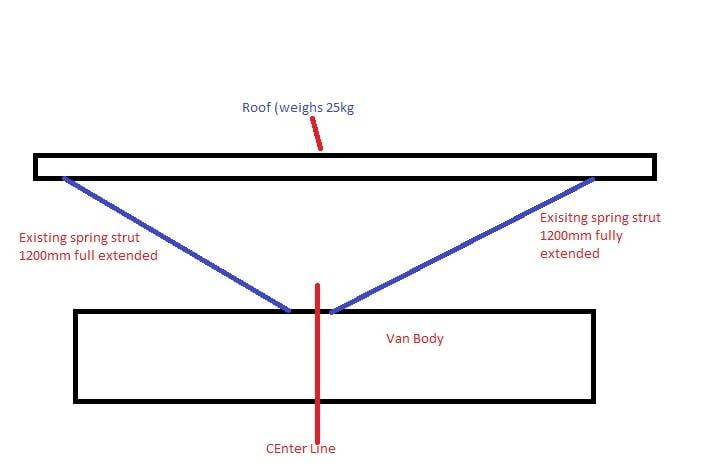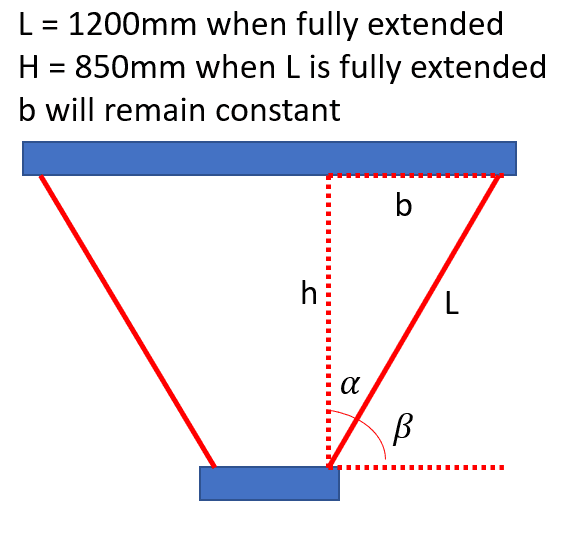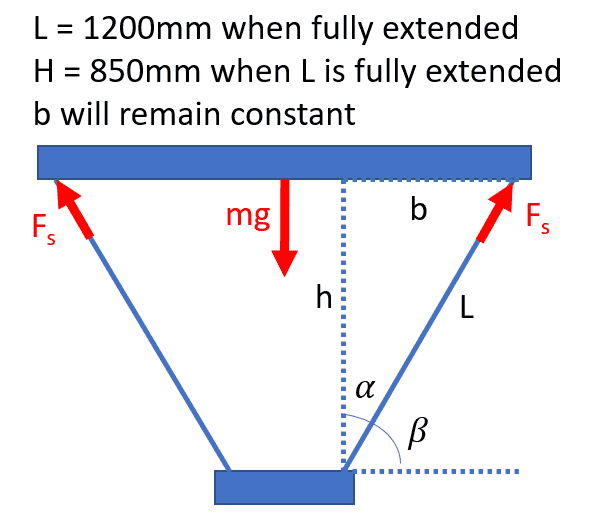# Force Required for Gas Struts on 1972 VW T2 Danbury Pop top campervan?

• I
• confused_steve
The length of the strut is 847mm when retracted and 1200mm when extended. The force needed to open the roof with the struts in this position is 98 newtons.f

#### confused_steve

TL;DR Summary
Campervan project, gas struts rating help needed
Hi all, i'm the proud owner of a 1972 VW T2 Danbury Pop top campervan. Out of the factory these were fitted with 4 x long spring loaded struts which (when new) provided some lifting power to pop the roof up. Mine are now 50 years old and provide no assistance lifting / holding the roof in its fully extended position.

I am looking to remove these 4 struts and replace with new gas struts. To be able to order the correct ones i need to determine the Newtons force per strut. I am looking for these struts to give good lifting assistance and then to hold the roof at its vertical capacity securely whilst not struggling to pull it back down again into its resting position on top of the van.

The roof weighs 25kg
The existing sprint struts measure 1200mm fully extended

I understand that to lift 25kg vertically it would take approx 250Nm of force, but how would i calculate accurately the force for my new gas struts that i want to install?

I was never great at physics at school ;)

I've attached an image of a side profile of the camper van showing some position / weight etc. Let me know if anyone needs anymore info. Thanks everyone!Welcome to PF.
It would help if we knew the height change of the roof.
How far does it lift?

Of course! Height change is 850mm

Thank you, i have found these previously. Unfortunately the nature of these campers is that over the last 50 years the canvas has probably been changed from standard spec and the roof had additional support / weight added which is why i'm looking to measure the needed force correctly and spec struts accordingly. They weren't willing to tell me what spec their struts were, i suspect because they'd know i'd go off and buy the struts at a total of £60 and not their £180 kit :)

Here is a first approximation.
When open, triangle height = 850 mm; strut length = 1200 mm.
That makes the strut angle close to 45°. √2 = 1.4142; 1/√2 = 0.7071;
When closed, the strut will be horizontal, and length will be close to 850 mm.

There are four struts supporting 25 kg + some tension in the fabric.
28/4 = 7 kg each.
A vertical 7 kg force, will require a strut at 45°, to give an axial 10 kg force.
A 10 kg force is 10 * 9.8 = 98 newtons.

Strut will be; 850 mm when retracted; 1200 mm when extended; force = 100 N.

•confused_steve
Here is a first approximation.
When open, triangle height = 850 mm; strut length = 1200 mm.
That makes the strut angle close to 45°. √2 = 1.4142; 1/√2 = 0.7071;
When closed, the strut will be horizontal, and length will be close to 850 mm.

There are four struts supporting 25 kg + some tension in the fabric.
28/4 = 7 kg each.
A vertical 7 kg force, will require a strut at 45°, to give an axial 10 kg force.
A 10 kg force is 10 * 9.8 = 98 newtons.

Strut will be; 850 mm when retracted; 1200 mm when extended; force = 100 N.
You sir are an absolute legend!! so 4 struts at 100N each should raise and support my roof!

Thank you!

•berkeman
Here is a first approximation.
When open, triangle height = 850 mm; strut length = 1200 mm.
That makes the strut angle close to 45°. √2 = 1.4142; 1/√2 = 0.7071;
When closed, the strut will be horizontal, and length will be close to 850 mm.

There are four struts supporting 25 kg + some tension in the fabric.
28/4 = 7 kg each.
A vertical 7 kg force, will require a strut at 45°, to give an axial 10 kg force.
A 10 kg force is 10 * 9.8 = 98 newtons.

Strut will be; 850 mm when retracted; 1200 mm when extended; force = 100 N.

I don't think that will be enough to open the roof. When fully extended the struts are loaded the least because they are pointing upwards which is aligned with the weight they must counteract. It would be necessary to study the worst-case scenario which is when the roof is fully closed so the struts will be more horizontal.The length ##b## is constant since it depends on the distance between the anchoring points for the struts. We can find its value from the information we know from the max aperture case.
##cos(\alpha_{min})=\frac{h_{max}}{L_{max}}\rightarrow \alpha_{min}=arcos(\frac{h_{max}}{L_{max}})##
Then, we can obtain the constant value of ##b## (the problem could be solved without it but using it makes everything clearer).
##L_{max}sin(\alpha_{min})=b\approx 847mm##
It is then possible to express the angle ##\alpha## as a function of ##h## or ##L## which are the changing parameters of the problem. I will choose ##h## because I consider it a more fundamental variable for the problem at hand.
##tan(\alpha)=\frac{b}{h} \rightarrow \alpha = atan(\frac{b}{h})##
For convenience in the following steps, I will define the angle ##\beta##.
##\beta =\frac{\pi }{4}-\alpha##
Once the geometry of the problem is clear I will analyze the forces acting on the roof. I will assume the acceleration of the roof is negligible since there is no point on open it quickly and it would only result in having greater loads. Also, although in the diagram there are only 2 struts, there actually are 4 symmetrical struts that are superposed because of the 2D representation.In the vertical direction ##(y)##we will have:
##\sum F_y=m\ddot{y}\rightarrow 4F_ssin(\beta)-mg=m\cancel{\ddot{y}}\rightarrow F_s=\frac{mg}{4sin(\beta)}##

As you can see from the previous equation, the force each strut needs to do goes to infinity as ##\beta\rightarrow 0^{+}##. This is something to be expected since we are trying to counteract the vertical force from the weight with nearly horizontal forces (so they have very small vertical components).

These sorts of systems are often designed so that the angle ##\beta## never becomes too small. For example, by lowering the anchor point at the bottom below the surface where the roof will reach the closed state.

To help OP with the design we would need a better description of the position of the anchoring points which I am sure will result in a geometry in which for ##h=0; \beta>>0##. Or to solve it more directly, if you have an approximation for the angle ##\beta## when the roof is closed it'd be enough to obtain some useful numbers for the necessary force from the struts.

As a reminder, the objective of the problem is to find the min length, max length, and max force necessary to open it (such an objective was defined by Baluncore when proposing a solution and it fully defines the system).

#### Attachments

•Baluncore and erobz
I am looking for these struts to give good lifting assistance and then to hold the roof at its vertical capacity securely whilst not struggling to pull it back down again into its resting position on top of the van.
As a reminder, the objective of the problem is to find the min length, max length, and max force necessary to open it (such an objective was defined by Baluncore when proposing a solution and it fully defines the system).
The zero angle when closed is not a problem, as the roof is raised and lowered by hand, probably one end at the time. The gas struts reduce the effort required, and support it in the open position while parked. The gas struts do not fight the straps or latches that hold the roof down while travelling.

This was not a clean-slate design problem, it was a low-cost strut replacement exercise.

••hutchphd, erobz and Juanda
The zero angle when closed is not a problem, as the roof is raised and lowered by hand, probably one end at the time. The gas struts reduce the effort required, and support it in the open position while parked. The gas struts do not fight the straps or latches that hold the roof down while travelling.

This was not a clean-slate design problem, it was a low-cost strut replacement exercise.

I am not familiar enough with these kind of roofs so I misunderstood what OP was asking for. It is much clearer to me now.

•Baluncore